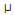When a silicon diode is forward biased, what is VBE for a C-E configuration?

What is the current gain for a common-base configuration where IE = 4.2 mA and IC = 4.0 mA?

A collector characteristic curve is a graph showing

If a 2 mV signal produces a 2 V output, what is the voltage gain?

The total emitter current is:

Which is the higher gain provided by a C-E configuration?

Often a common-collector will be the last stage before the load; the main function(s) of this stage is to:

What is the collector current for a C-E configuration with a beta of 100 and a base current of 30A?

The input/output relationship of the common-collector and common-base amplifiers is:

If VCC = +18 V, voltage-divider resistor R1 is 4.7 kΩ, and R2 is 1500Ω, what is the base bias voltage?